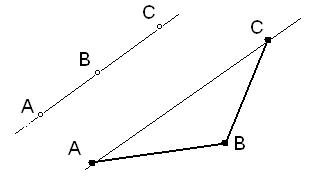### Calculate Collinearity of Three Points

Calculate Collinearity for given 3-points A, B, C and tells points are collinear or non-collinear.
Three or more points are said to be collinear if they lie on a single straight line. The condition for three points A, B, and C to be collinear can also be expressed as the distance between any one point and the line determined by the other two is zero means resultant value is equal to zero, then the points are collinear otherwise points are non-collinear and if points are non-collinear then a triangle can be formed with these three points as vertices.Point A (x1,y1) = Point B (x2,y2) = Point C (x3,y3) =